×#### Thank you for registering.

One of our academic counsellors will contact you within 1 working day.

Click to Chat

1800-1023-196

+91-120-4616500

CART 0

• 0

MY CART (5)

Use Coupon: CART20 and get 20% off on all online Study Material

ITEM
DETAILS
MRP
DISCOUNT
FINAL PRICE
Total Price: Rs.

There are no items in this cart.
Continue Shopping• Complete JEE Main/Advanced Course and Test Series
• OFFERED PRICE: Rs. 15,900
• View Details

```Chapter 7: Statistics Exercise – 7.1

Question: 1

Calculate the mean for the following distribution:

x:
5
6
7
9
9

f:
4
8
14
11
3

Solution:

x
f
fx

5
4
20

6
8
48

7
14
98

8
11
88

9
3
27

N = 40
281

Mean = 281/40 =7.025

Question: 2

Find the mean of the following data:

x:
19
21
23
25
27
29
31

f:
13
15
16
18
16
15
13

Solution:

x
f
fx

18
13
247

21
15
315

23
16
368

25
18
450

27
16
432

29
15
435

31
13
403

N = 106
Sum = 2620

Mean (x) = 2680/106 = 25

Question: 3

If the mean of the following data is 20.6. Find the value of p.

x:
10
15
P
25
35

f:
3
10
25
7
5

Solution:

x
f
fx

10
3
30

5
10
150

P
25
25p

25
7
175

35
5
175

N = 50
Sum = 2620 + 25P

Given Mean = 20.6

20.6 = (530 + 25p)/50

20.6 25p = 20.6 P

P = 20

Question: 4

If the mean of the following data is 15, find p

x:
5
10
15
20
25

f:
6
p
6
10
5

Solution:

x
f
fx

5
6
30

10
P
10p

15
6
90

20
10
200

25
5
125

N = p + 27
Sum = 10p + 445

Given Mean =1515P + 405 = 10P + 445

15P - 10P = 445-405

5P = 40

P = 5

Question: 5

Find the value of p for the following distribution whose mean is 16.6

x:
8
12
15
p
20
25
30

f:
12
16
20
24
16
8
4

Solution:

x
f
fx

8
12
96

12
12
192

15
20
300

P
24
24p

20
16
320

25
8
200

30
4
120

N = 100
Sum = 24p + 1228

Given Mean = 16.6

16.6 = (24p +1228)/100

1660  =  24p + 1228

24p = 1660 – 1228

P = 432/24

P = 18

Question: 6

Find the missing value of p for the following distribution whose mean is 12.58

x:
5
8
10
12
p
20
25

f:
2
5
8
22
7
4
2

Solution:

x
f
fx

5
2
10

8
5
40

10
8
80

12
22
264

P
7
7p

20
24
480

25
2
50

N = 50
Sum = 524 + 7p

Given mean = 12.58
Mean = Sum/N
12.58 =  (524 +7p)/50
629 = 524 + 7p
629 - 524 = 7p
105 = 7P
P = 105/7
P = 15

Question: 7

Find the missing frequency (p) for the following distribution whose mean is 7.68.

x:
3
5
7
9
11
13

f:
6
8
15
p
8
4

Solution:

x
f
fx

3
6
18

5
8
40

7
15
105

9
p
9p

11
8
88

13
4
52

N = P + 41
Sum =  9p + 303

Given Mean = 7.68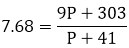7.68 (P + 41) = 9P + 303
7.68 P + 314.88 = 9P + 303
9P – 7.68 P = 314.88 – 303
1.32 P = 11.88
P = 11.88/1.32
P = 9

Question: 8

The following table gives the number of boys of a particular age in a class of 40 students. Calculate the mean age of the students.

Ages (in years):
15
16
17
18
19
20

No of students:
3
8
10
10
5
4

Solution:

x
f
fx

15
3
45

16
8
128

17
10
170

18
10
180

19
5
95

20
4
80

N = 40
Sum = 698

Mean age = sum/ N
= 698/ 40
= 17.45 years

Question: 9

Candidates of four schools appear in a mathematics test. The data were as follows:

Schools
No of candidates
Average score

I
60
75

II
48
80

III
P
55

IV
40
50

If the average score of the candidates of all the four schools is 66, find the number of candidates that appeared from school III.

Solution:

Let the number candidates from school III = P

Schools
No of candidates Ni
Average scores (xi)

I
60
75

II
48
80

III
P
55

IV
40
50

Given Average score or all schools = 66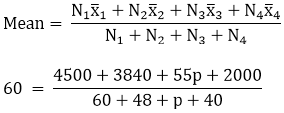10340 + 55p = 66 p + 9768
10340 - 9768 = 66p –55 p
P = 572/11
P = 52

Question: 10

Five coins were simultaneously tossed 1000 times and at each toss, the number of heads was observed. The number of tosses during which 0, 1, 2, 3, 4 and 5 heads were obtained are shown in the table below. Find the mean number of heads per toss.

No of tosses

0
38

1
144

2
342

3
287

4
164

5
25

Total
1000

Solution:

No of tosses

0
38

1
144

2
342

3
287

4
164

5
25

Total
1000

No of tosses
fx

0
38
0

1
144
144

2
342
684

3
287
861

4
164
656

5
25
125

Mean number of heads per toss = 2470/1000
= 2.47
Mean = 2.47

Question: 11

The arithmetic mean of the following data is 25. Find the value of k.

x
5
15
25
35
45

f
3
k
3
6
2

Solution:

x
f
fx

5
3
15

15
k
15k

25
3
75

35
6
210

45
2
90

N = k + 14
Sum = 15k + 390

Given mean = 25
Sum/ N = 2525K + 350 = 15K + 390
25 K – 15K = 390 - 350
10 k = 40
K = 40/10
K = 4

Question: 12

If the mean of the following data is 18.75. Find the value of p.

x
10
15
p
25
30

f
5
10
7
8
2

Solution:

x
f
fx

10
5
50

15
10
150

20
p
20p

25
8
200

30
2
60

N = P + 25
Sum = 20p + 460

Given mean = 18.75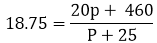18.75p + 468.75 = 20p + 460
468.75 – 460 = 20p – 18.75p
8.75 = 1.25P
P = 8.75/1.25
P = 7

Question: 13

Find the value of p. If the mean of the following distribution is 20.

x
15
17
19
20 + p
23

f
2
3
4
5p
6

Solution:

x
f
fx

15
2
30

17
3
51

19
4
76

20 + p
5p
100p + 5p2

23
6
138

N = 5p + 15`
Sum = 295 +100p +5p2

Given Mean = 20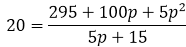100p + 300 = 295 + 100p + 5p2
300 – 295 = 5p2 + 100p
5 = 5p2 + 100P
5p2 + 100P – 5 =0
5p2 + 20p –5P
5(p2 – 1)  = 0
P2 – 1 = 0
P (+1, -1)
If p + 1 = 0, p = -1, Or p – 1 = 0, P = 1

Question: 14

Find the missing frequencies in the following frequency distribution if it is known that the mean of the distribution is 50. If ∑f = 120

x
10
30
50
70
90

f
17
f1
32
f2
19

Solution:

x
f
fx

10
17
170

30
f1
30f1

50
32
1600

70
f2
70f2

90
19
1710

N = 68 + f1 + f2
Sum = 30f1 + 70f2 + 3480

Given mean = Sum/N
= 50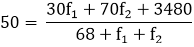3400 + 50 f1 + 50 f2 = 30 f1 + 70 f2 + 3480
50 f1 – 30 f1 + 50 f2 – 70 f2 = 3480 – 3400
20 f1 – 20 f2 = 80
f1 – f2 = 4    ….. (i)
f1 + f2 + 68 = 120
f1 + f2 = 52 …. (ii)
f1 = 28
f2 = 24
```### Course Features

• 728 Video Lectures
• Revision Notes
• Previous Year Papers
• Mind Map
• Study Planner
• NCERT Solutions
• Discussion Forum
• Test paper with Video Solution